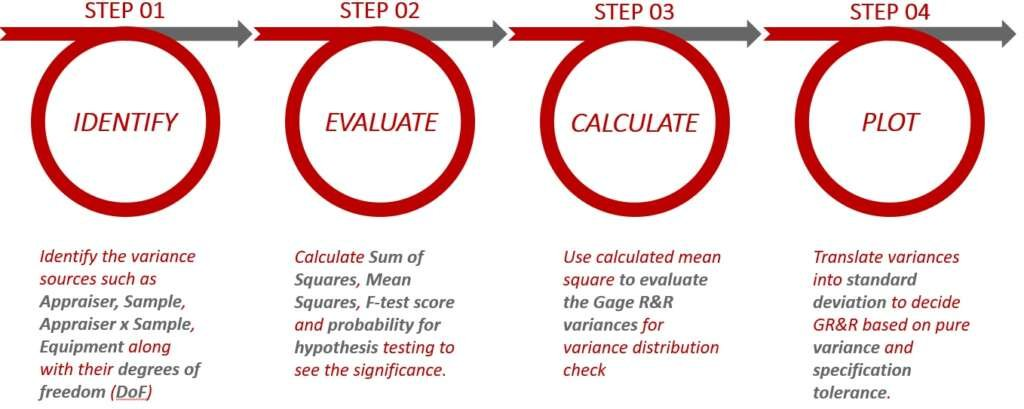# 37. MSA – Gage R&R ANOVA Method

This section will detail the procedures and steps of how to calculate measurement system’s gage R&R via Analysis of Variance method (ANOVA).

In the measurement system, these are the main variances coming from sources which are listed below.

However, measurements factors coming from the main variance is not solely the contribution. Because interactions between cross factors such as repeated sample’s measurement by individual appraiser will occur (as in MSA Type II study). Therefor ANOVA is required to analyze any potential interaction and verify the significance between the interaction of appraiser and samples.

For the ANOVA Gage R&R setup, please refer to the following process flow to understand the overall sequence of evaluation steps.ANOVA Gage R&R Calculation Steps

In order to begin the ANOVA calculation, the identification of variance source is required before calculating the gage R&R. The table below will illustrate the equations for the indicators before calculating gage R&R. For the example this section will apply, 4 appraisers will be measuring 12 different samples with total of 4 measurements per sample.ANOVA Calculation Setup Equation

Throughout the calculation, the only difficulties to calculate would be the reliability and total variance. The toggle section will explain the details of each sum square which involves with summation notation. While the p-value indicates the significant level for the factor to impact interaction level inside the measurement system.

Square the differences between appraiser’s average and grand overall average. Then add it up before multiplying with number of trials and number of samples

Square the differences between sample’s average and grand overall average. Then add it up before multiplying with number of trials and number of appraisers.

Square the differences between each measurement point (in this case there are 192 data points (4 x 4 x 12) and grand overall average. Then add it up together for the total of sum square.

Square the differences between each part’s trial value and their respective part’s overall trial average by the particular operator. Then add it up together for the total of sum square in equipment.

By looking at the excel sheet below, the highlighted blue cell in the left is used to calculate the differences between each data point with grand overall averages (calculating SS for total) while the light purple cells are used to store data for appraiser’s trial averages per sample before calculating the differences of each measurement for calculating sum square of repeatability.ANOVA Calculation Data Cell

After the ANOVA constant table is calculated, then it will be the final steps to convert the Gage R&R factors. Please refer to the following table for each calculated result along with its equation.ANOVA Gage R&R Constant Calculation and Representation

Before making the verdict for the measurement system, please refer to the following table for the criteria acceptance for both the variance contribution and standard deviation’s contribution.ANOVA Variance % Contribution Acceptance Standard.ANOVA Standard Deviation’s % Contribution Acceptance

The following image contains the calculated result based on the ANOVA approach.ANOVA Finalized Calculation Result & Description of Measurement System.